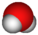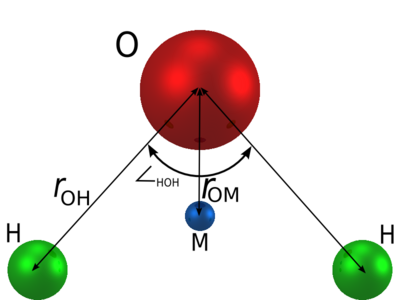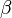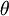# TIP4P/2005f water modelThis article is a 'stub' about water and/or ice. It has no, or next to no, content. It is here at the moment to help form part of the structure of SklogWiki. If you add material to this article, remove the {{Stub-water}} template from this page.$r_{\mathrm {OH}}$ (Å)$\angle$HOH , deg$\sigma$ (Å)$\epsilon/k$ (K) q(O) (e) q(H) (e) q(M) (e)$r_{\mathrm {OM}}$ (Å) Dr (kJ/mol)$\beta$ (1/nm) K($\theta$) (kJ/(mol rad^2)) 0.9419 107.4 3.1644 93.2 0 0.5564 -2q(H) 0.1546 432.581 22.87 367.810